Download PresentationGlucose (Inside)

# Glucose (Inside) - PowerPoint PPT Presentation

= S. KINETICS OF PASSIVE GLUCOSE TRANSPORT INTO (AND OUT OF) CELLS. S e (extracellular). Glucose (Inside). S i (Intracellular). k -1. k 1. Suspend cells in glucose solution for time t.I am the owner, or an agent authorized to act on behalf of the owner, of the copyrighted work described.
Download Presentation## Glucose (Inside)

An Image/Link below is provided (as is) to download presentation

Download Policy: Content on the Website is provided to you AS IS for your information and personal use and may not be sold / licensed / shared on other websites without getting consent from its author.While downloading, if for some reason you are not able to download a presentation, the publisher may have deleted the file from their server.

- - - - - - - - - - - - - - - - - - - - - - - - - - E N D - - - - - - - - - - - - - - - - - - - - - - - - - -
Presentation Transcript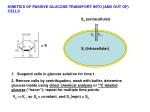= S

KINETICS OF PASSIVE GLUCOSE TRANSPORT INTO (AND OUT OF) CELLS

Se (extracellular)

Glucose (Inside)

Si (Intracellular)

k-1

k1

• Suspend cells in glucose solution for time t

2. Remove cells by centrifugation, wash with buffer, determine glucose inside using direct chemical analysis or 14C labeled glucose (“tracer”); repeat for multiple time points.

Ve >>Vi , so Se  constant, and Si (eqm) = Se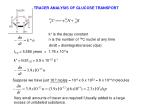TRACER ANALYSIS OF GLUCOSE TRANSPORT

14

k* is the decay constant

n is the number of 14C nuclei at any time

dn/dt = disintegrations/sec (dps)

t1/2 = 5,580 years = 1.76 x 1011s

k* = ln2/t1/2 = 3.9 x 10-12 s-1

Suppose we have just 10-9 moles = 10-9 x 6 x 1023 = 6 x 1014 molecules

Very small amounts of tracer are required! Usually added to a large excess of unlabeled substance.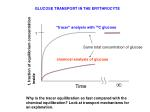GLUCOSE TRANSPORT IN THE ERYTHROCYTE

“tracer” analysis with 14C glucose

1

Same total concentration of glucose

f, fraction of equilibrium concentration

inside

chemical analysis of glucose

Why is the tracer equilibration so fast compared with the chemical equilibration? Look at transport mechanisms for an explanation.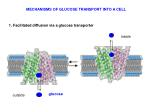MECHANISMS OF GLUCOSE TRANSPORT INTO A CELL

1. Facilitated diffusion via a glucose transporter

inside

glucose

outside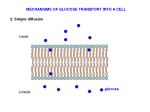MECHANISMS OF GLUCOSE TRANSPORT INTO A CELL

2. Simple diffusion

inside

glucose

outside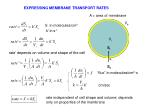“flux” in molecules/cm2-s

cm/sec

EXPRESSING MEMBRANE TRANSPORT RATES

A = area of membrane

Ve

S in molecules/cm3

k’ in s-1

Vi

Si

rate’ depends on volume and shape of the cell

k

Se

rate independent of cell shape and volume; depends only on properties of the membrane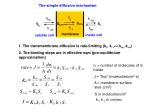The simple diffusion mechanism

1. The transmembrane diffusion is rate-limiting (k1, k-1<< km, k-m)

2. The binding steps are in effective eqm (pre-equilibrium approximation)

ni = number of molecules of S inside

J = “flux” (molecules/cm2-s)

A = membrane surface area (cm2)

S in molecules/cm3

k1, k-1 in cm/sec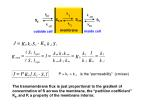P = k1 = k-1 is the “permeability” (cm/sec)

The transmembrane flux is just proportional to the gradient of concentration of S across the membrane, the “partition coefficient” Kb,and P, a property of the membrane interior.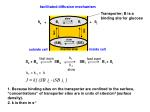k1

fast eqm

fast eqm

Se + Be SBe SBi Bi + Si

k-1

slow

facilitated diffusion mechanism

Transporter; B is a binding site for glucose

k1 = k-1 = k

1. Because binding sites on the transporter are confined to the surface, “concentrations” of transporter sites are in units of sites/cm2 (surface density).

2. k is then in s-1k1

fast eqm

fast eqm

Se + Be SBe SBi Bi + Si

k-1

slow

Eliminate concentrations of intermediates (pre-equilibrium approx)

Conservation of transporter sites on each surface; BTi and BTe are the total concentrations of binding sites at the respective surfaces

Transporter sites randomly distributed between surfaces; BT is total conentration of sites at each surface.

After a little algebra (done in appendix 1)

Facilitated diffusionsimple

facilitated

Initial rates (Si = 0)

Jmax = kBTwhen Se >> Km

Initial rate behavior clearly distinguishes simple from facilitated diffusion mechanisms

In terms of JmaxTHE EXPERIMENTAL RESULT WE ARE TRYING TO EXPLAIN

“tracer” analysis with 14C glucose

1

f, fraction of equilibrium concentraiton

inside

chemical analysis of glucose

To see if this behavior can be accounted for by either mechanism, we will express the rate equations in terms of f, the fraction of equilibrium concentration inside the cell (the y axis on the plot)(Se does not change much during approach to equilibrium)

ne

Define the fractional approach to equilibrium, f

ni

Vin

Vout

Vout >> Vin

For simple diffusion

Jmax is at f = 0

Jmax = PKbSeFacilitated diffusion

Divide numerator and denominator of flux equation by Sefacilitated diffusion

Simple diffusion

Relative flux at Km/Se = 0.1 (transporter sites saturated)

Only halfway to equilibrium, the flux of the facilitated diffusion has dropped to 8% of its maximum value, while the simple diffusion continues at 50% of its maximum. Why is the facilitated process so slow here?

f = Si/(Si)eqmSe/Km

This result can account for the anomalous rapid equilibration of “tracer” glucose while the chemical equilibration is slow. The tracer is in very low concentration, well below saturation. In this case, the efflux is not saturated, and much slower than the influx. Although the net flux is rather low due to low Se, it does not have far to go to reach equilibrium. This is most easily seen by determining the time dependence of f.Vi = cell internal volume

Si in molecules/cm3

Divide through by Se

f = Si/Se

Where r = radius of a spherical cell

Can be solved numerically for f = f(t) for particular choices of Se, Km, r, BT, kSpherical cell of r = 5mm

Km = 1x10-3 Molar

k= 200 molecules s-1

3/r = A/V = 6 x 103 cm-1

BT = 1011 transporters cm2

(surface/volume of cell)

Tracer experiment has very low effective Se! Even in the presence of a large excess of unlabeled glucose, the equilibration of tracer is fast.

f = Si/Se = Si/(Si)eqm

Chemical analysis experiment has high Se(1)

Let ye and yi be the fractions of carrier sites at each surface that are complexed with S:

(2)

Where BTe and BTi are the total concentrations of binding sites at the external and internal surfaces, respectively, ie

(3)

Thus,

(4)

Now define the dissociation constant of S from the carrier as

(5)

On rearrangement

(6)Substitution of Be, Bi from (6) into (4)

(7)

Using (7) and (2)

(8)

Substituting (8) into (1)

(9)

If we assume that the binding sites on the transporter are randomly distributed between the two faces of the membrane

(10)

Finally, (9) and (10) giveFor 14C decay,

1Curie (Ci) = 3.7 x 1010 dps

You can buy 14C glucose with 1mCi/mole “specific activity”

Make a solution of 0.1 M 14C glucose

In one liter, there are 0.1 Mole x 1mCi/mole = 0.1 mCi = 10-4 Ci of 14C glucose

10-4Ci x 3.7 x 1010 dps/Ci = 3.7 x 106 dps

n  1 x 1018 molecules of 14C glucose

Moles of 14C glucose = 1 x 1018 / 6 x 1023 = 1.7 x 10-6

Therefore, the concentration of 14C glucose is only 1.7 x 10-6 Molar in the 0.1Molar glucose solution. The actual tracer concentration is very low.